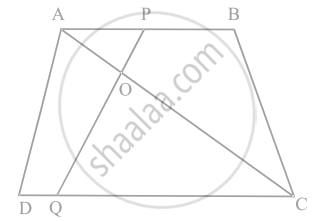# In figure, if AB || DC and AC, PQ intersect each other at the point O. Prove that OA . CQ = OC . AP - Mathematics

Sum

In figure, if AB || DC and AC, PQ intersect each other at the point O. Prove that OA . CQ = OC . AP#### Solution

AC and PQ intersect each other at the point O and AB || DC.

From ∆AOP and ∆COQ,

∠AOP = ∠COQ   ......[Since they are vertically opposite angles]

∠APO = ∠CQO   .....[Since, AB || DC and PQ is transversal, the angles are alternate angles]

∴ ∆AOP ∼ ∆COQ  ......[Using AAA similarity criterion]

Then, since, corresponding sides are proportional

We have,

(OA)/(OC) = (AP)/(CQ)

OA × CQ = OC × AP

Hence Proved.

Concept: Similarity of Triangles
Is there an error in this question or solution?

#### APPEARS IN

NCERT Mathematics Exemplar Class 10
Chapter 6 Triangles
Exercise 6.3 | Q 5 | Page 67
Share